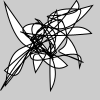# bezier_vertices()#

Create a collection of bezier vertices.

## Examples#import numpy as np

def setup():
random_bezier_vertices = 100 * np.random.rand(25, 6)
py5.begin_shape()
py5.vertex(py5.width / 2, py5.height / 2)
py5.bezier_vertices(random_bezier_vertices)
py5.end_shape()


## Description#

Create a collection of bezier vertices. The purpose of this method is to provide an alternative to repeatedly calling bezier_vertex() in a loop. For a large number of bezier vertices, the performance of bezier_vertices() will be much faster.

The coordinates parameter should be a numpy array with one row for each bezier vertex. The first few columns are for the first control point, the next few columns are for the second control point, and the final few columns are for the anchor point. There should be six or nine columns for 2D or 3D points, respectively.

Underlying Processing method: bezierVertices

## Signatures#

bezier_vertices(
coordinates: npt.NDArray[np.floating],  # 2D array of bezier vertex coordinates with 6 or 9 columns for 2D or 3D points, respectively
/,
) -> None


Updated on March 06, 2023 02:49:26am UTC